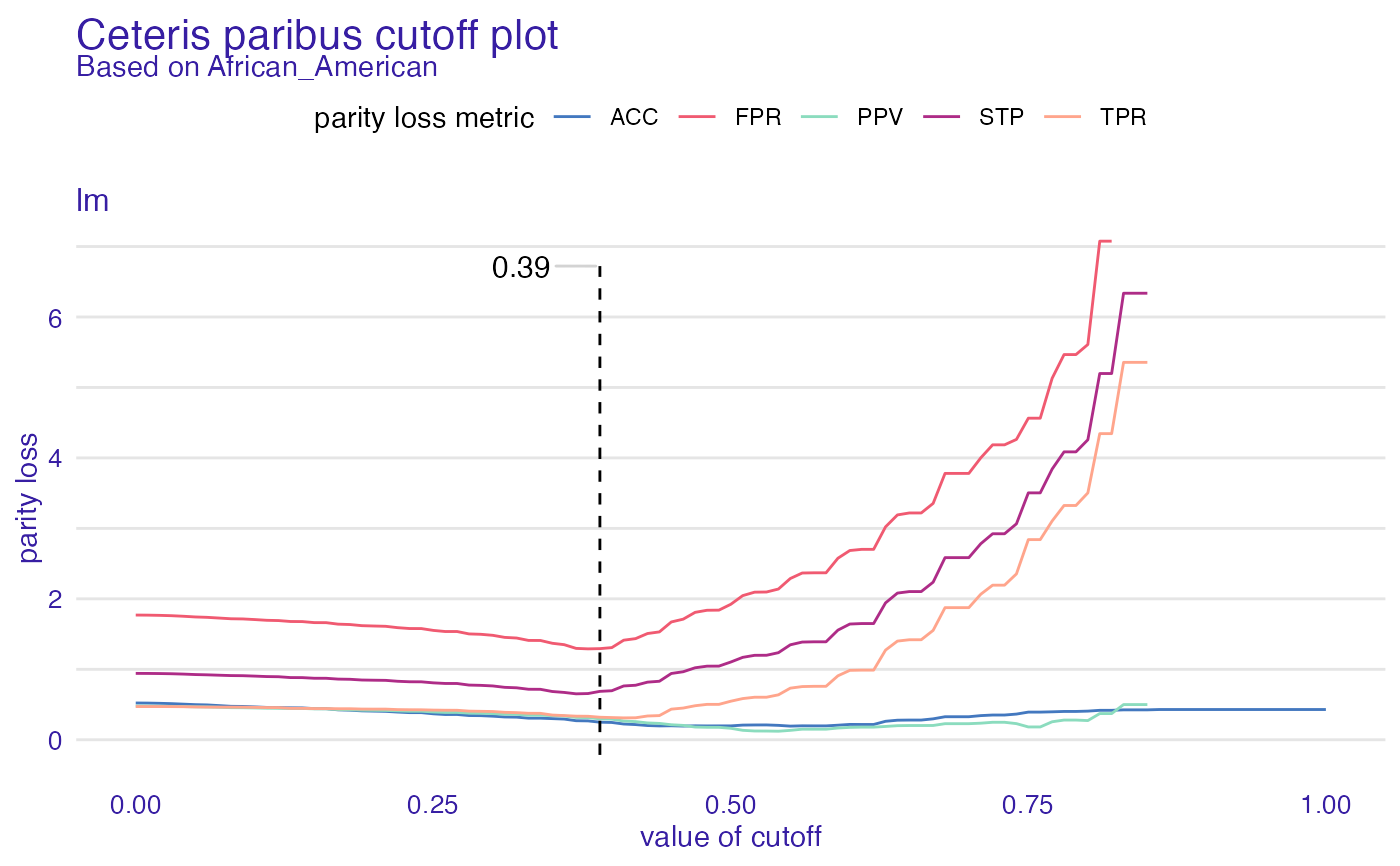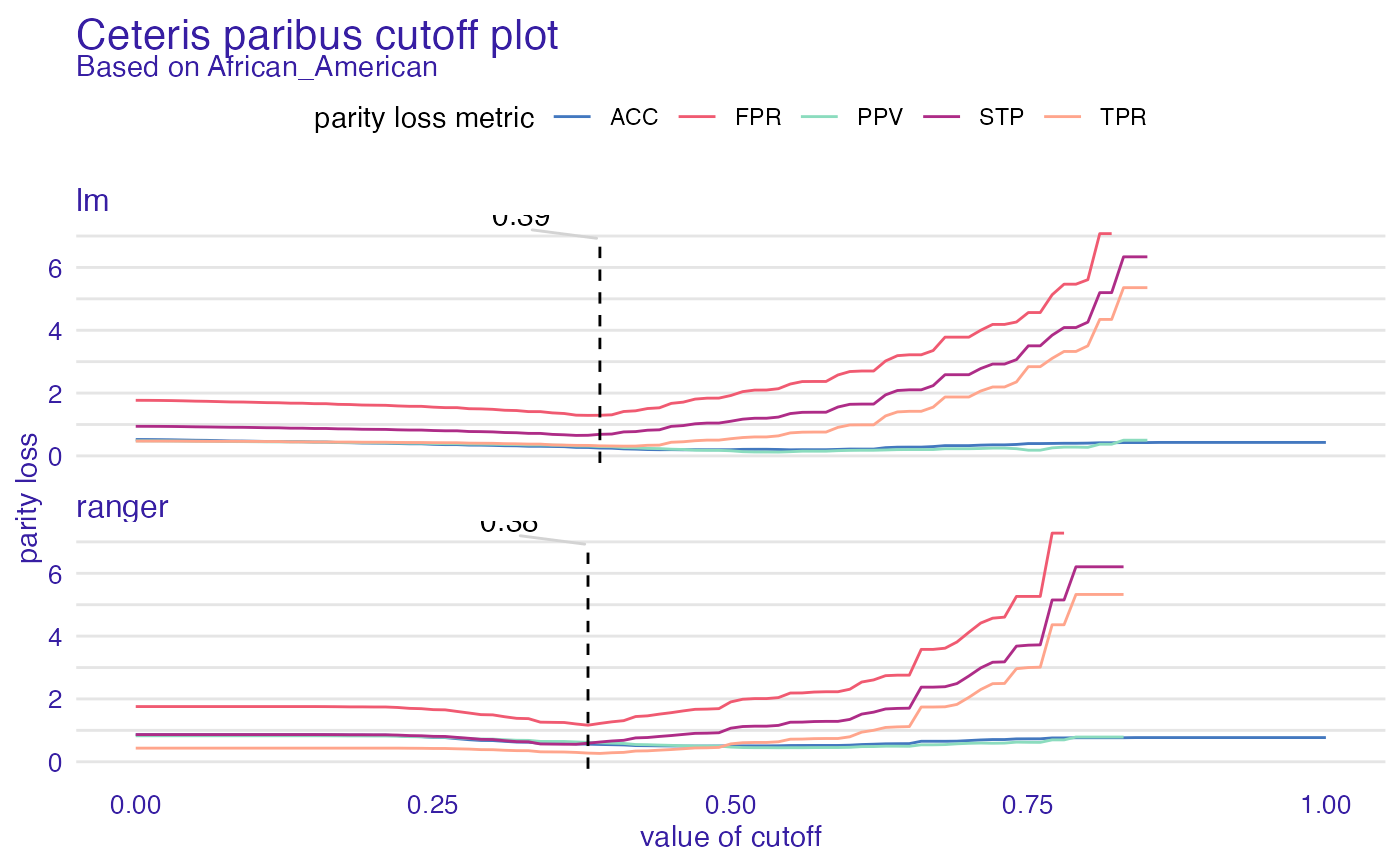Ceteris paribus cutoff is way to check how will parity loss behave if we changed only cutoff in one subgroup. It plots object of class ceteris_paribus_cutoff. It might have two types - default and cumulated. Cumulated sums metrics and plots it all in one plot. When default one is used all chosen metrics will be plotted for each model.

# S3 method for ceteris_paribus_cutoff
plot(x, ...)

Arguments

x ceteris_paribus_cutoff object other plot parameters

ggplot2 object

Examples

data("compas")

# positive outcome - not being recidivist
two_yr_recidivism <- factor(compas$Two_yr_Recidivism, levels = c(1, 0)) y_numeric <- as.numeric(two_yr_recidivism) - 1 compas$Two_yr_Recidivism <- two_yr_recidivism

lm_model <- glm(Two_yr_Recidivism ~ .,
data = compas,
)

explainer_lm <- DALEX::explain(lm_model, data = compas[, -1], y = y_numeric)
#> Preparation of a new explainer is initiated
#>   -> model label       :  lm  (  default  )
#>   -> data              :  6172  rows  6  cols
#>   -> target variable   :  6172  values
#>   -> predict function  :  yhat.glm  will be used (  default  )
#>   -> predicted values  :  No value for predict function target column. (  default  )
#>   -> model_info        :  package stats , ver. 4.1.1 , task classification (  default  )
#>   -> predicted values  :  numerical, min =  0.004522979 , mean =  0.5448801 , max =  0.8855426
#>   -> residual function :  difference between y and yhat (  default  )
#>   -> residuals         :  numerical, min =  -0.8822826 , mean =  -5.07018e-13 , max =  0.9767658
#>   A new explainer has been created!

fobject <- fairness_check(explainer_lm,
protected = compas$Ethnicity, privileged = "Caucasian" ) #> Creating fairness classification object #> -> Privileged subgroup : character ( Ok ) #> -> Protected variable : factor ( Ok ) #> -> Cutoff values for explainers : 0.5 ( for all subgroups ) #> -> Fairness objects : 0 objects #> -> Checking explainers : 1 in total ( compatible ) #> -> Metric calculation : 11/13 metrics calculated for all models ( 2 NA created ) #> Fairness object created succesfully cpc <- ceteris_paribus_cutoff(fobject, "African_American") plot(cpc) #> Warning: Removed 63 row(s) containing missing values (geom_path).# \donttest{ rf_model <- ranger::ranger(Two_yr_Recidivism ~ ., data = compas, probability = TRUE, num.trees = 200 ) explainer_rf <- DALEX::explain(rf_model, data = compas[, -1], y = y_numeric) #> Preparation of a new explainer is initiated #> -> model label : ranger ( default ) #> -> data : 6172 rows 6 cols #> -> target variable : 6172 values #> -> predict function : yhat.ranger will be used ( default ) #> -> predicted values : No value for predict function target column. ( default ) #> -> model_info : package ranger , ver. 0.13.1 , task classification ( default ) #> -> predicted values : numerical, min = 0.153945 , mean = 0.5452013 , max = 0.8650518 #> -> residual function : difference between y and yhat ( default ) #> -> residuals : numerical, min = -0.847902 , mean = -0.0003212319 , max = 0.7775046 #> A new explainer has been created! fobject <- fairness_check(explainer_lm, explainer_rf, protected = compas$Ethnicity,
privileged = "Caucasian"
)
#> Creating fairness classification object
#> -> Privileged subgroup		: character ( Ok  )
#> -> Protected variable		: factor ( Ok  )
#> -> Cutoff values for explainers	: 0.5 ( for all subgroups )
#> -> Fairness objects		: 0 objects
#> -> Checking explainers		: 2 in total (  compatible  )
#> -> Metric calculation		: 8/13 metrics calculated for all models ( 5 NA created )
#>  Fairness object created succesfully

cpc <- ceteris_paribus_cutoff(fobject, "African_American")
plot(cpc)
#> Warning: Removed 73 row(s) containing missing values (geom_path).# }